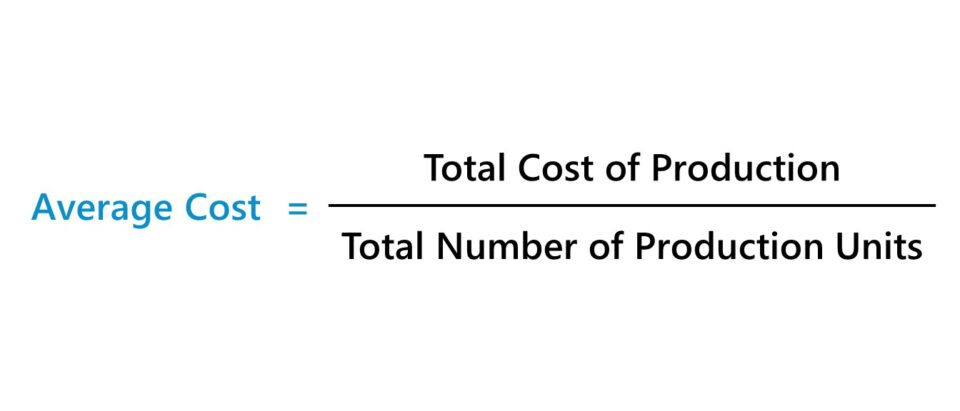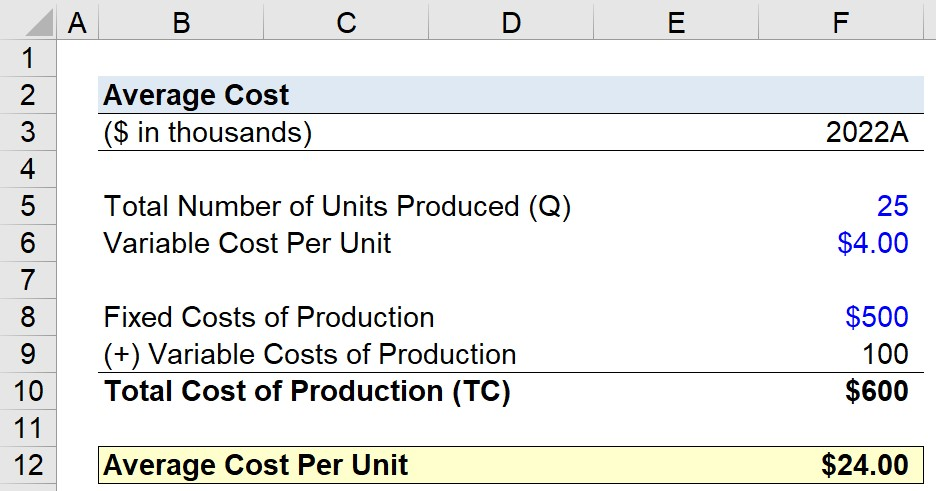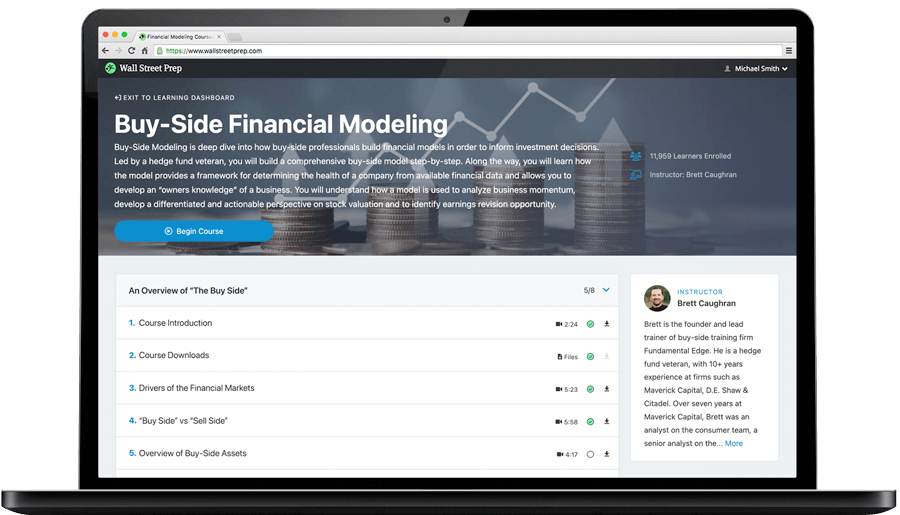Welcome to Wall Street Prep! Use code at checkout for 15% off.# Average Cost (Per Unit Cost)

Guide to Understanding the Average Cost ("Per Unit Cost")## How to Calculate Average Cost (Step-by-Step)

The average cost represents the standard cost incurred per unit of production.

The calculation of the average cost is relatively straightforward, since the per-unit cost represents the ratio between the total cost of production and the total number of production units.

The importance of measuring the average cost is tied to setting the pricing of products appropriately, wherein enough profits are generated per sale for the company’s operations to remain sustainable.

In theory, the price point at which a good is sold should be based on the current state of the market conditions (i.e. supply and demand), which is a fundamental part of researching a particular market, target customer profile, and business model optimization.

The process of determining a company’s average cost is as follows.

• Step 1 → Count the Total Number of Units Produced in a Given Period
• Step 2 → Determine the Total Production Costs Incurred in the Corresponding Period
• Step 3 → Divide the Total Cost of Production by the Total Number of Units Produced

## Average Cost Formula (Per Unit Cost)

The formula to calculate the average cost is as follows.

Average Cost = Total Cost of Production ÷ Total Number of Units Produced

Broadly, the total cost of production is composed of two parts, as expressed by the following formula.

Total Cost of Production = Fixed Costs + Variable Costs

While the total cost of production is often abbreviated as “TC”, the total number of units produced is frequently denoted as “Q”.

## Marginal Cost Analysis: Fixed Cost vs. Variable Cost Per Unit

The total cost of production can be segmented into two distinct types of costs, which are differentiated by their relationship (or lack thereof) with the volume of output.

1. Fixed CostFixed costs remain the same irrespective of the production volume, i.e. they are independent of the unit output.
2. Variable Cost→ Variable costs fluctuate based on the output of production, i.e. an increase in production volume coincides with higher variable costs (and vice versa).

Initially, the average cost tends to decline as more units are produced—i.e. economies of scale—but the cost savings and benefits to a company’s profit margins reverse course beyond a certain threshold in terms of unit production output.

Near the aforementioned inflection point, most of the incurred incremental per-unit costs are of variable nature, rather than fixed costs. Therefore, companies must establish set internal targets regarding the number of units to produce (and sell to the market) in order to operate at a level where profitability is near maximized.

Otherwise, the positive effects of economies of scale start to diminish as the marginal benefit derived from the production of each incremental unit declines (and the marginal cost per unit causes profits to decline).

• Marginal Cost < Average Cost: Increased Production Output → Lower Average Cost
• Marginal Cost > Average Cost: Decreased Production Output → Higher Average Cost

## Average Cost Calculator – Excel Template

We’ll now move on to a modeling exercise, which you can access by filling out the form below.Submitting...

## Average Cost Calculation Example (Per-Unit Cost)

Suppose an OEM equipment manufacturer produced a total of 25,000 parts and components in the fiscal year ending 2022.

Amid the conversion of raw materials it purchased into finished goods ready to be sold to its customers, the manufacturer incurred a total of \$500,000 in fixed costs.

• Total Number of Units Produced (Q) = 25,000
• Total Fixed Costs = \$500,000

Moreover, the variable cost per unit of production is \$4.00, so the total variable costs incurred over the course of the fiscal year were \$100,000.

• Variable Cost Per Unit = \$4.00
• Total Variable Costs = \$4.00 × 25,000 = \$100,000

The sum of the manufacturer’s fixed and variable costs, i.e. the total cost of production, comes out to \$600,000.

• Total Cost of Production (TC) = \$500,000 + \$100,000 = \$600,000

In the final step of our exercise, the total cost of production is divided by the total quantity of units produced to arrive at an average cost of \$24.00.

• Average Cost Per Unit = \$600,000 ÷ 25,000 = \$24.00Step-by-Step Online Course

### Learn Buy-Side (Hedge Fund) Modeling

Led by a former hedge fund PM (Maverick, Citadel, DE Shaw, Schonfeld), this program begins where financial modeling training ends — with a deep-dive into how buy-side analysts build financial models to make key investment decisions.